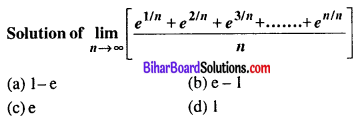# Bihar Board 12th Maths VVI Objective Questions Model Set 1 in English

Bihar Board 12th Maths Objective Questions and Answers

## Bihar Board 12th Maths VVI Objective Questions Model Set 1 in English

Question 1.
Let A = {5, 6}; how many binary operations can be defined on this set?
(a) 8
(b) 10
(c) 16
(d) 20
(c) 16

Question 2.
Let A = {1, 2, 3}, which of the following functions f : A → A does not have an inverse function?
(a) {(1, 1), (2, 2), (3, 3)}
(b) {(1, 2), (2, 1), (3, 1)}
(c) {(1, 3), (3, 2), (2, 1)}
(d) {(1, 2), (2, 3), (3, 1)}
(b) {(1, 2), (2, 1), (3, 1)}

Question 3.
If A = {1, 2, 3}, B = {6, 7, 8} and f : A → B is a function such that f(x) = x + 5 then what type of a function is f?
(a) into
(b) one-one onto
(c) many-one onto
(d) Constant function
(b) one-one onto

Question 4.
What type of a relation is “Less than” in the set of real numbers?
(a) only symmetric
(b) only transitive
(c) only reflexive
(d) equivalence relation
(b) only transitive

Question 5.(a) $$\frac{8 \pi}{5}$$

Question 6.
cos-1(2x – 1) =
(a) 2cos-1 x
(b) cos-1 √x
(c) 2cos-1 √x
(d) None of these
(c) 2cos-1 √x

Question 7.
2cot-1 3 + cot-1 7 =
(a) $$\frac{\pi}{2}$$
(b) π
(c) $$\frac{\pi}{4}$$
(d) $$\frac{\pi}{6}$$
(b) π

Question 8.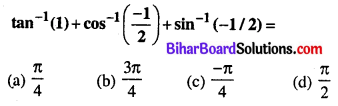(b) $$\frac{3 \pi}{4}$$

Question 9.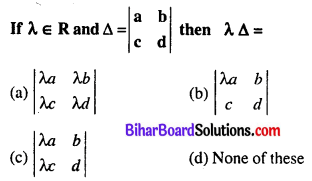(a) $$\left|\begin{array}{ll} \lambda a & \lambda b \\ \lambda c & \lambda d \end{array}\right|$$

Question 10.
If a, b, c are in A.P. then :
$$\left|\begin{array}{ccc} x+1 & x+2 & x+a \\ x+2 & x+3 & x+b \\ x+3 & x+4 & x+c \end{array}\right|=$$
(a) 3
(b) -3
(c) 0
(d) 1
(c) 0

Question 11.
If 7 and 2 are two roots of the equation $$\left|\begin{array}{lll} x & 3 & 7 \\ 2 & x & 2 \\ 7 & 6 & x \end{array}\right|=0$$ then the third root is :
(a) -9
(b) 14
(c) $$\frac{1}{2}$$
(d) None of these
(a) -9

Question 12.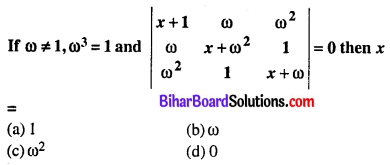(d) 0

Question 13.(b) $$\frac{\pi}{3}$$

Question 14.
If A be a square matrix. Then A + A’ will be a _________
(a) Symmetric matrix
(b) Skew symmetric matrix
(c) Null matrix
(d) Unit matrix
(a) Symmetric matrix

Question 15.
If A is a matrix of order 3 × 3, such that A2 = A then (A + I3)3 – 7A is equal to?
(a) I3
(b) A
(c) 3A
(d) I3 – A
(a) I3

Question 16.
Let A be a non-singular matrix of the order 2 × 2 then |adj A| = ________
(a) 2|A|
(b) |A|
(c) |A|2
(d) |A|3
(b) |A|

Question 17.
$$\frac{d}{d x}$$[log(sec x + tan x)] =
(a) $$\frac{1}{\sec x+\tan x}$$
(b) sec x
(c) tan x
(d) sec x + tan x
(b) sec x

Question 18.
If x2y3 = (x + y)5 then $$\frac{d y}{d x}=$$
(a) $$\frac{x}{y}$$
(b) $$\frac{y}{x}$$
(c) $$\frac{-y}{x}$$
(d) $$\frac{-x}{y}$$
(b) $$\frac{y}{x}$$

Question 19.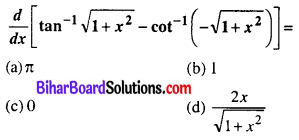(c) 0

Question 20.(c) $$\left(\frac{2}{3}\right)^{x} \log _{3} 2$$

Question 21.
f(x) = √3 sin x + cos x is maximum then value of x = ______
(a) $$\frac{\pi}{6}$$
(b) $$\frac{\pi}{2}$$
(c) $$\frac{\pi}{3}$$
(d) $$\frac{\pi}{4}$$
(c) $$\frac{\pi}{3}$$

Question 22.
If y = log cos x2, then x = √π at $$\frac{d y}{d x}$$ has the value :
(a) 1
(b) $$\frac{\pi}{4}$$
(c) 0
(d) √π
(c) 0

Question 23.
Equation of the tangent to the curve x2 + y2 = a2 at (x1, y1) is :
(a) xx1 – yy1 = 0
(b) xx1 + yy1 = 0
(c) xx1 – yy1 = a2
(d) xx1 + yy1 = a2
(d) xx1 + yy1 = a2

Question 24.
$$\frac{d}{d x}\left[\lim _{x \rightarrow a} \frac{x^{5}-a^{5}}{x-a}\right]=$$
(a) 5a4
(b) 5x4
(c) 1
(d) 0
(a) 5a4

Question 25.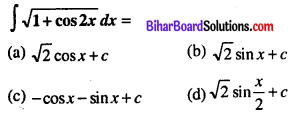(b) √2 sin x + c

Question 26.(b) $$\frac{1}{3} e^{x^{3}}+c$$

Question 27.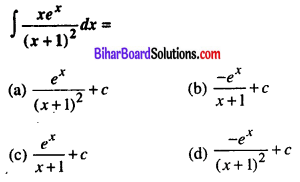(a) $$\frac{e^{x}}{(x+1)^{2}}+c$$

Question 28.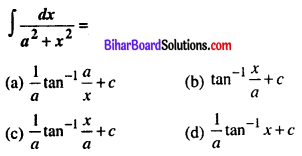(c) $$\frac{1}{a} \tan ^{-1} \frac{x}{a}+c$$

Question 29.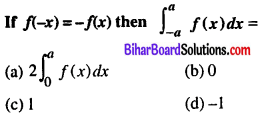(b) 0

Question 30.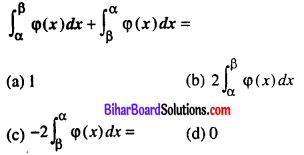(d) 0

Question 31.
Area between the x-axis and the curve y = sin x, from x = 0 to x = $$\frac{\pi}{2}$$ is :
(a) 2
(b) -1
(c) 1
(d) None of these
(c) 1

Question 32.
$$\int_{0}^{1}(x) d x=\dots$$
(a) 0
(b) 1
(c) 2
(d) $$\frac{1}{2}$$
(d) $$\frac{1}{2}$$

Question 33.
The differential equation $$1+\left(\frac{d y}{d x}\right)^{2}=\left(\frac{d^{2} y}{d x^{2}}\right)^{3}$$ is of order = _______ and degree = _______
(a) order = 2, degree = 3
(b) order = 1, degree = 2
(c) order = 2, degree = 2
(d) None of these
(a) order = 2, degree = 3

Question 34.
Solution of the differential equation y dx – x dy = xy dx is
(a) $$\frac{y^{2}}{2}-\frac{x^{2}}{2}=x y+c$$
(b) x = kyex
(c) x = kyey
(d) None of these
(b) x = kyex

Question 35.
Integrating factor (I.F.) of differential equation $$\frac{d y}{d x}+\frac{y}{x}=\frac{y^{2}}{x^{2}}$$ is
(a) log x
(b) x
(c) $$\frac{1}{x}$$
(d) None of these
(c) $$\frac{1}{x}$$

Question 36.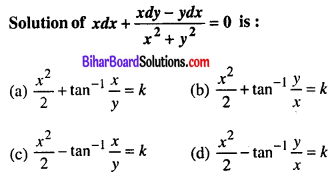(b) $$\frac{x^{2}}{2}+\tan ^{-1} \frac{y}{x}=k$$

Question 37.(b) $$\frac{\vec{i}+\vec{j}+2 \vec{k}}{\sqrt{6}}$$

Question 38.(b) $$\frac{3}{13}, \frac{-4}{13}, \frac{12}{13}$$

Question 39.
$$x \vec{i}-3 \vec{j}+5 \vec{k},-x \vec{i}+x \vec{j}+2 \vec{k}$$ are perpendicular to each other then value of x =
(a) -2, 5
(b) 2, 5
(c) -2, -5
(d) 2, -5
(d) 2, -5

Question 40.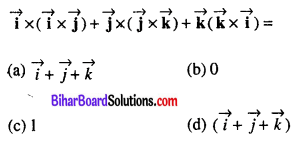(b) 0

Question 41.
The direction Cosines of y axis are :
(a) (1, 0, 1)
(b) (0, 1, 0)
(c) $$\left(\frac{1}{\sqrt{2}}, 0, \frac{1}{\sqrt{2}}\right)$$
(d) None of these
(b) (0, 1, 0)

Question 42.
The equation of the xy plane is :
(a) x = 0, y = 0
(b) z = 0
(c) x = y ≠ 0
(d) None of these
(b) z = 0

Question 43.
If two planes 2x – 4y + 3z = 5 and x + 2y + λz = 12 are perpendicular to each other, then a =
(a) -2
(b) 2
(c) 3
(d) None of these
(b) 2

Question 44.
The distance bewteen (4, 3, 7) and (1, -1, 5) is :
(a) 13
(b) 15
(c) 12
(d) 5
(a) 13

Question 45.
If A’ and B’ are independent events then :
(a) P(A’B’) = P(a) . P(b)
(b) P(A’B’) = P(A’) + P(B’)
(c) P(A’B’) = P(A’) . P(B’)
(d) P(A’B’)= P(AP) – P(B’)
(c) P(A’B’) = P(A’) . P(B’)

Question 46.
If events A and B are mutually exclusive then :
(a) P(A∩B) = P(a) . P(b)
(b) P(A∩B) = 0
(c) P(A∩B) = 1
(d) P(A∪B) = 0
(b) P(A∩B) = 0

Question 47.(c) $$\frac{2}{3}$$

Question 48.
If A and B are two events such that P(A) ≠ 0 and $$\mathbf{P}\left(\frac{\mathbf{B}}{\mathbf{A}}\right)=\mathbf{1}$$
(a) B ⊂ A
(b) A ⊂ B
(c) B = φ
(d) A∩B = φ
(b) A ⊂ B

Question 49.
$$\int \frac{d x}{x+\sqrt{x}} d x$$
(a) log x + log(1 + √x) + C
(b) 2log(1 + √x) + C
(c) log(1 + √x) + C
(d) log√x + C